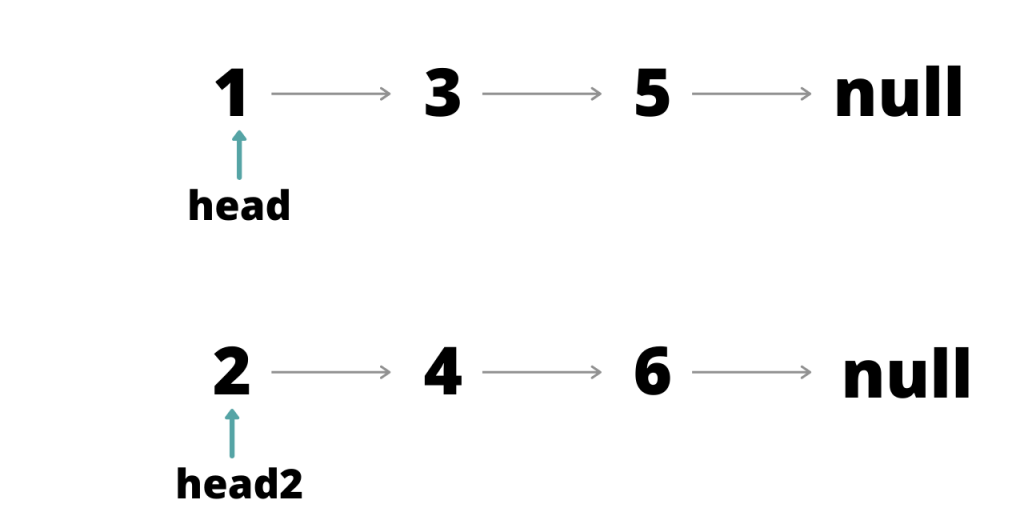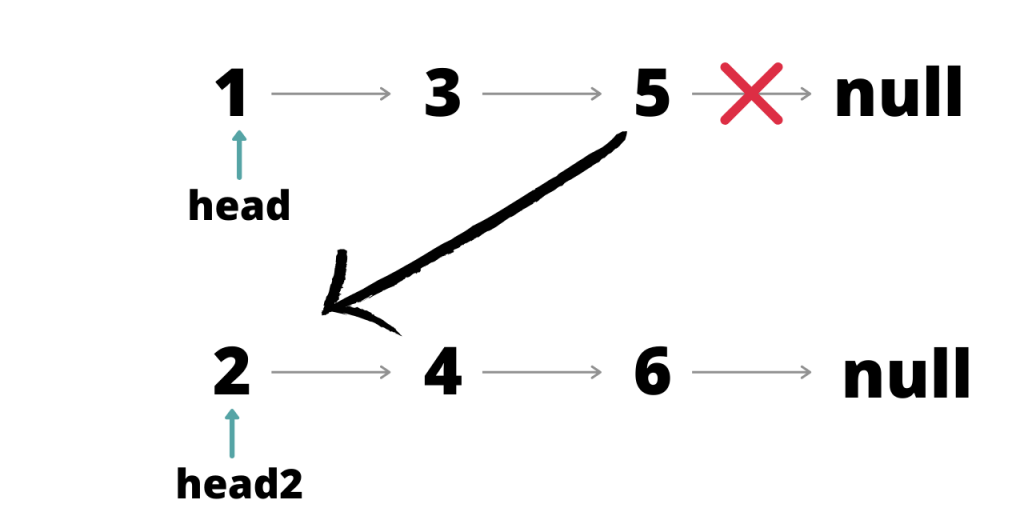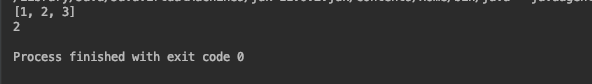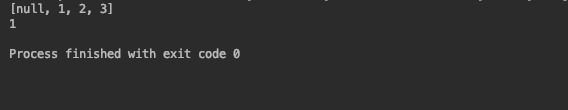tag:www.digitalocean.com,2005:/community/tags/list.atom DigitalOcean Community | List tutorials, questions and resources 2023-01-27T19:24:20.358Z DigitalOcean <![CDATA[How to Merge Two Lists in Java?]]> tag:www.digitalocean.com,2005:/community/tutorials/merge-two-lists-in-java 2022-08-03T19:33:38.005Z Merging two lists in Java is often a useful operation. These lists can be ArrayLists or LinkedLists.

## How to Merge Two Lists in Java

There are multiple ways we can merge two lists in Java. Let’s explore some of the straightforward ones to get your job done!

### 1. The addAll() method to merge two lists

The addAll() method is the simplest and most common way to merge two lists.

For ArrayList :

``````import java.util.ArrayList;

public class Main {

public static void main(String[] args)
{
ArrayList<Integer> l1 = new ArrayList<Integer>();
ArrayList<Integer> l2 = new ArrayList<Integer>();
ArrayList<Integer> merge = new ArrayList<Integer>();
System.out.println("L1 : "+l1);
System.out.println("L2 : "+l2);
System.out.println("Merged : "+merge);
}
}
``````

Note the order of appearance of elements matches the order in which addAll() is called.

``````import java.util.LinkedList;

public class Main {

public static void main(String[] args)
{

System.out.println("L1 : "+L1);
System.out.println("L2 : "+L2);
System.out.println("Merged : "+merged);
}
}
``````

### 2. Using iterators to merge two lists in Java

We can use an Iterator to traverse the list and merge.

For ArrayList :

``````import java.util.ArrayList;

public class Main {

public static void main(String[] args)
{
ArrayList<Integer> l1 = new ArrayList<Integer>();
ArrayList<Integer> l2 = new ArrayList<Integer>();

ArrayList<Integer> Itmerge = new ArrayList<>();
Iterator i = l1.iterator();
while (i.hasNext()) {
}
i=l2.iterator();
while (i.hasNext()) {
}
System.out.println("L1 : "+l1);
System.out.println("L2 : "+l2);
System.out.println("Merged : "+Itmerge);
}
}
``````

Iterator first traverses the ArrayList l1 and adds all the elements to Itmerge, it then traverses the ArrayList l2 and adds all the elements to Itmerge.

Another way to merge the two lists is to simply add elements from one list to the other. There is no need to create a new list unless you need to keep the existing data intact.

``````Iterator i = l1.iterator();
while (i.hasNext())
{
}

System.out.println("Merged : "+l2);
``````

In this case, all the elements are added to the list l2. This saves the memory spent in the creation of an extra list. Adding the elements of one list to another saves the extra traversal.

``````import java.util.LinkedList;

public class Main {

public static void main(String[] args)
{

Iterator i = L1.iterator();
while (i.hasNext()) {
}
System.out.println(L2);
}
}
``````### 3. Merge Multiple Lists using for loop

For loop is also useful to merge two lists.

For ArrayList:

``````import java.util.ArrayList;

public class Main {

public static void main(String[] args)
{
ArrayList<Integer> l1 = new ArrayList<Integer>();
ArrayList<Integer> l2 = new ArrayList<Integer>();
ArrayList<Integer> Itmerge = new ArrayList<>();
for(int i=0;i<l1.size();i++){
}
for(int i=0;i<l2.size();i++){
}
System.out.println(Itmerge);
}
}
``````

The loop traverses both the ArrayLists and adds each element one by one to a newly created list.

Adding the elements of one list to another saves the extra traversal.

`````` for(int i=0;i<l2.size();i++){
}
System.out.println(l1);
``````

This for loop adds the elements of l2 to l1 one by one. In this case, l1 will contain the final list of merged elements.

To understand the traversal of linked lists a little better let us define our own linked list.

This will require a class for nodes. A node needs two things, data and the address of the next node.

Code for class node :

``````public class node {
int data;
node next;
public node(int data){
this.data=data;
next=null;
}
}
``````

Note that the next is of type node since it stores the address of a node. Creating the same two lists as used in earlier examples:

``````public class Main {

public static void main(String[] args)
{
node temp = new node(3);
node temp1 = new node(5);
temp.next=temp1;
node temp2 = new node(4);
node temp3 = new node(6);
temp2.next=temp3;
}
}
``````

This will create lists that look like :Each arrow represents the next link. To link the two lists together we need to link the end of one list to the head of the second.This can be done as follows:

``````node trav=head;
while(trav.next!=null){
trav=trav.next;
}
``````

A node ‘trav’ is initiated and pointed at the head of the first list. The first list is traversed until trav reaches the end of the first list.

When the end is reached, it changes the next link of the last node to head of the second list. This forms a link between the two lists.

Printing all the lists:

``````public class Main {

public static void main(String[] args)
{
node temp = new node(3);
node temp1 = new node(5);
temp.next=temp1;
node temp2 = new node(4);
node temp3 = new node(6);
temp2.next=temp3;

//printing list 1
System.out.println("List 1 :");

while(trav!=null){
System.out.print(trav.data + " ");
trav=trav.next;
}

System.out.println();
//prinitng list 2
System.out.println("List 2 :");

while(trav!=null){
System.out.print(trav.data + " ");
trav=trav.next;
}
System.out.println();

//merging the two list

while(trav.next!=null){
trav=trav.next;
}
// printing merged list
System.out.println("merged list :");
while(trav!=null){
System.out.print(trav.data + " ");
trav=trav.next;
}
}
}
``````

## Conclusion

We saw the different ways to merge two lists in Java. These are ranged from inbuilt functions to basic for loops. The last example above gives a deeper understanding of how lists work in Java. Using the approach of the last example you can have more control over the order in which elements appear in the list.

]]>
Jayant Verma 2022-08-03T19:33:38.005Z
<![CDATA[How to Convert Set to List in Java]]> tag:www.digitalocean.com,2005:/community/tutorials/set-to-list-in-java 2022-08-03T17:52:17.919Z Lists in Java are ordered collection of data, whereas sets are an unordered collection of data. A list can have duplicate entries, a set can not. Both the data structures are useful in different scenarios.

Knowing how to convert a set into a list is useful. It can convert unordered data into ordered data.

## Initializing a set

Let’s initialize a set and add some elements to it.

``````import java.util.*;

public class Main {

public static void main(String[] args)
{
Set<Integer> a = new HashSet<>();
System.out.println(a);
}
}
``````The add() method of HashSet adds the elements to the set. Note that the elements are distinct. There is no way to get the elements according to their insertion order as the sets are unordered.

## Converting Set to List in Java

Let’s convert the set into a list. There are multiple ways of doing so. Each way is different from the other and these differences are subtle.

### 1. List Constructor with Set argument

The most straightforward way to convert a set to a list is by passing the set as an argument while creating the list. This calls the constructor and from there onwards the constructor takes care of the rest.

``````import java.util.*;

public class Main {

public static void main(String[] args)
{
Set<Integer> a = new HashSet<>();

List<Integer> arr = new ArrayList<>(a);
System.out.println(arr);
System.out.println(arr.get(1));

}
}
``````

Since the set has been converted to a list, the elements are now ordered. That means we can use the get() method to access elements by index.

### 2. Using conventional for loop

We can use the good old for loop to explicitly copy the elements from the set to the list.

``````import java.util.*;

public class Main {

public static void main(String[] args)
{
Set<Integer> a = new HashSet<>();

List<Integer> arr = new ArrayList<>(a);
for (int i : a)
System.out.println(arr);
System.out.println(arr.get(1));
}
}
``````For loop goes over the set element by element and adds the elements to the list.

Lists have a method called addAll() that adds multiple values to the list at once. You might recall this operation from its use in merging two lists. addAll() also works for adding the elements of a set to a list.

``````import java.util.*;

public class Main {

public static void main(String[] args)
{
Set<Integer> a = new HashSet<>();

List<Integer> arr = new ArrayList<>();
System.out.println(arr);
System.out.println(arr.get(1));
}
}
``````### 4. Stream API collect() method

Stream.collect() is available from Java 8 onwards. ToList collector collects all Stream elements into a List instance.

``````import java.util.*;
import java.util.stream.Collectors;

public class Main {

public static void main(String[] args)
{
Set<Integer> a = new HashSet<>();

List<Integer> arr;

arr = a.stream().collect(Collectors.toList());
System.out.println(arr);
System.out.println(arr.get(1));
}
}
``````The documentation for stream.collect() mentions that there is no guarantee on the type, mutability, serializability, or thread-safety of the List returned. If more control over the returned List is required, use toCollection(Supplier).

To specify the type of list use toCollection(ArrayList::new)

``````import java.util.*;
import java.util.stream.Collectors;

public class Main {

public static void main(String[] args)
{
Set<Integer> a = new HashSet<>();

List<Integer> arr;

arr = a.stream().collect(Collectors.toCollection(ArrayList::new));
System.out.println(arr);
System.out.println(arr.get(1));
}
}
``````

### 5. List.copyOf() method

Java 10 onwards List has a copyOf() method. The method returns an unmodifiable List containing the elements of the given Collection, in its iteration order. The list can’t contain any null elements. In case the set contains ‘null’, the method returns a null pointer exception.

``````import java.util.*;
import java.util.stream.Collectors;

public class Main {

public static void main(String[] args)
{
Set<Integer> a = new HashSet<>();

List<Integer> arr;

arr = List.copyOf(a);
System.out.println(arr);
System.out.println(arr.get(1));
}
}
``````Adding a null in the set and trying to convert to a list :

``````import java.util.*;
import java.util.stream.Collectors;

public class Main {

public static void main(String[] args)
{
Set<Integer> a = new HashSet<>();

List<Integer> arr;

arr = List.copyOf(a);
System.out.println(arr);
System.out.println(arr.get(1));
}
}
``````

If you try to add an element to an immutable list you’ll get an error that looks like the following.

Using the addAll() method to convert set with null element to a list :

``````import java.util.*;
import java.util.stream.Collectors;

public class Main {

public static void main(String[] args)
{
Set<Integer> a = new HashSet<>();

List<Integer> arr = new ArrayList<>();

//  arr = List.copyOf(a);
System.out.println(arr);
System.out.println(arr.get(1));
}
}
``````Note that the null appears at the beginning of the list.

## Conclusion

We saw some really interesting methods to convert a set into a list. It’s important to pay attention to the type of list that is created from each method. Like copyOf() method produces an immutable list and can’t handle null elements. Whereas, stream.collect() doesn’t guarantee anything. Constructor and addAll() are the most trustworthy among the batch.

]]>
Jayant Verma 2022-08-03T17:52:17.919Z